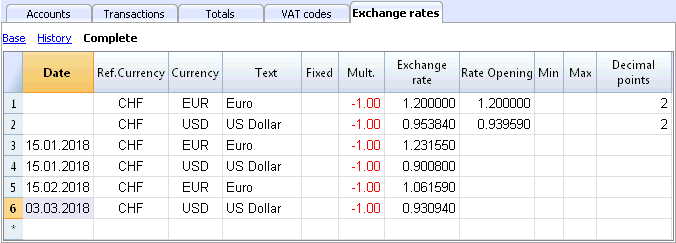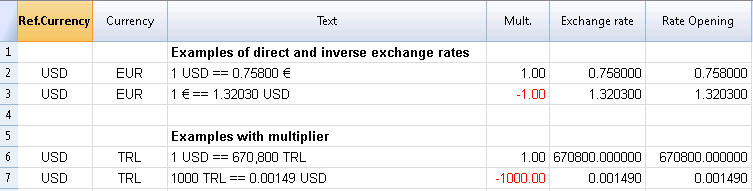# Exchange rates table

Before entering multi-currency transactions it is necessary to define the parameters of the used currencies in the Exchange rates table.## Columns of the Exchange rate table

Date
The date for the exchange rate.

• Exchange rate rows without date. Actual and Opening exchange rate.
For each used foreign currency, it is necessary to have a row in the Exchange rate table with an exchange rate and without a date.

• Exchange rate
This is the one that is considered to be the most recent and also the closing exchange rate.
It is being used for the calculation of the Balance Calculated column (Accounts table) and always when there is no historical exchange rate.
• Rate Opening
It is being used to convert the opening balance amounts of foreign currencies into the opening balance amounts of the basic currency of the accounting.
• Rows with a date (historical exchange rates)
The program chooses the exchange rate with a the date equal to the transaction row or with the closest preceding date to that row, in the following cases:

• If you have an historical exchange rate, the value in the Exchange rate column will be used as default exchange rate when you insert a new transaction
• When the exchange rate differences transactions are created and the option to use the historical exchange rate is activated
• When entering an exchange rate with a date, the Opening exchange rate is not used.

Ref. Currency
This is the currency that serves as the basis for the exchange rate (the CHF in our example.)

Currency
This is the destination currency, the one into which the value of the “Ref. Currency” will be converted.

Text
A text to indicate the foreign currency that we are dealing with.

Fixed
True or false. If there is a fixed exchange rate, enter Yes in this column. The used exchange rate is specified in the Exchange Rate column.

Mult.
The multiplier is usually 1, 100 or 1000 and is used to obtain the effective exchange rate. The multiplier is used for currencies which have a very low unit value in order to avoid having to insert exchange rates with many zeros. The multiplier can also be negative (-1). In this case, the program will use an inverted exchange rate or else it will act as if the currencies inserted in the Currency and Reference Currency columns had actually been inverted. Do not alter the multiplier once there are already transactions in the same currency, otherwise the program will signal a transaction error due to erroneous exchange rates.

Exchange rate
This column shows the actual exchange rate or the closing exchange rate for the currency compared to the reference currency.
It is also being used for calculating the exchange rate differences. Before calculating the exchange rate differences or closing the accounting, this value must be updated by entering the closing exchange rate.

The exchange rate and the multiplier are being applied according to the following formulas

• With multiplier > 0
Currency amount = Ref. currency amount* (exchange rate / |mult.|)

• With multiplier < 0
Ref. currency amount = Currency amount * (exchange rate / |mult.|)

Opening Exchange rate
This is the exchange rate at the moment the accounting is opened. To be indicated only on a row without date.

• It is used to convert the opening amount of the foreign currency into the opening amount of the accounting’s basic currency.
• Should be corresponding to the closing exchange rate of the preceding year.
If the closing exchange rates are not equal to the opening exchange rates, the Assets and Liabilities might result into different totals; see Differences in the Opening Balances.
• The opening exchange rate should never be changed in the course of the year, otherwise exchange rate differences are being created in the total of the opening balances.
• When creating a new year or when updating the opening balances, the program defines the opening exchange rate with the value indicated in the Exchange rate column (row without date) of the accounting of the previous year.

Minimum
This column shows the minimum exchange rate accepted. If a lesser exchange rate is used during the entry, there will be a warning.

Maximum
This column shows the maximum exchange rate accepted. If a greater exchange rate is used during the entry, there will be a warning.

Decimal Points
This column shows the number of decimal points to be used when rounding the amounts in currency2.

## Modifications in the Exchange rate table

The exchange rates entered in the transactions are standalone and independent of the exchange rates shown in the Exchange rate table. If you change an exchange rate in the Exchange rate table, there will be no repercussions in the transactions already submitted.

However, the following modifications do have repercussions:
• Modification of the Opening exchange rate
The next time you recalculate the accounting, the balances in basic currency of the accounts will be recalculated with the new exchange rate. Therefore, pay attention when modifying the Opening exchange rates once you have inserted the opening balances.
If you modify the Opening exchange rates and there are opening balances, it is important to recalculate the accounting.
• Modifying the multiplier
• When changing the multiplier of a currency already used in the Transactions table, the program will report a warning as soon as the accounting is being recalculated or will move to the transaction row.
The transaction amount and the correct amount in the basic currency will have to be reentered.
• When the accounting is being recalculated, as a result the opening balances in basic currency will be recalculated.

## Direct an indirect exchange rates

• Direct exchange rates are those where the basic currency and the foreign currency are being indicated on the same row.
In the example below there are direct exchange rates USD->EUR and USD->TRL.
• Indirect exchange rates are those where a direct exchange between two currencies is not being indicated (not recommended).
The exchange rate is deducted by the programn based on other combinations of entered exchage rates
In this example, the EUR->TRL exchange rate has not been defined, and the program relies on combining the USD-EUR and USD-TRL.
The program supports indirect exchange rates. Howver, we recommend using only direct exchange rates. Avoid the use of accounts where the direct exchange rate between the basic currency and the currency of the account is not specified. With indirect exchanges, it might not always be clear which exchange rate is being applied.## Historical exchange rates - exchange rate history

As explained above, if an exchange has a date, the program considers it to be a historical exchange rate; the program chooses the exchange rate with a date equal to the transaction row or with the closest preceding date to that row, in the following cases:

• If you have an historical exchange rate, the value in the Exchange rate column will be used as default exchange rate when you insert a new transaction
• When the exchange rate differences transactions are created and the option to use the historical exchange rate is activated
• When entering an exchange rate with a date, the Opening exchange rate is not used.You can import changes from another .ac2 file or copy / paste historical exchange rates from Excel for example.
If you import several historical change rates at the end of your accounting period, that is when you have already entered accounting transactions, to make sure that the program uses the imported exchange rates instead of those entered in the transactions simply manually delete the transactions exchange rates (Exchange rate column, Transactions table).